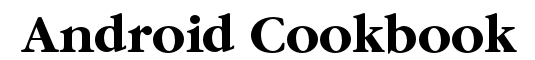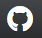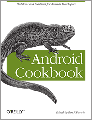A Crowd-sourced Cookbook on Writing Great Android® Apps### Using Pinch to Zoom

In Chapter: Graphics
Author: Pratik Rupwal ('prupwal')
Published? true
FormatLanguage: WikiFormat

### Problem:

Use the finger touch to change the position of an image viewed on the screen and use pinch-in and pinch-out movements for zooming in and zooming out operations.

### Solution:

Scale the image as a matrix to apply transformations to it for showing different visual effects.

### Discussion:

A simple ImageView is to be added inside a FrameLayout in main.xml as below:

```<?xml version="1.0" encoding="utf-8"?>
<FrameLayout
xmlns:android="http://schemas.android.com/apk/res/android"
android:layout_width="fill_parent"
android:layout_height="fill_parent" >
<ImageView android:id="@+id/imageView"
android:layout_width="fill_parent"
android:layout_height="fill_parent"
android:src="@drawable/nature"
android:scaleType="matrix" >
</ImageView>
</FrameLayout>
```

The code below scales the ImageView as a matrix to apply transformations on it.

```import android.app.Activity;
import android.graphics.Bitmap;
import android.graphics.Matrix;
import android.graphics.PointF;
import android.os.Bundle;
import android.util.FloatMath;
import android.util.Log;
import android.view.MotionEvent;
import android.view.View;
import android.view.View.OnTouchListener;
import android.widget.GridView;
import android.widget.ImageView;

public class Touch extends Activity implements OnTouchListener {
private static final String TAG = "Touch";

// These matrices will be used to move and zoom image
Matrix matrix = new Matrix();
Matrix savedMatrix = new Matrix();

// We can be in one of these 3 states
static final int NONE = 0;
static final int DRAG = 1;
static final int ZOOM = 2;
int mode = NONE;

// Remember some things for zooming
PointF start = new PointF();
PointF mid = new PointF();
float oldDist = 1f;

@Override
public void onCreate(Bundle savedInstanceState) {
super.onCreate(savedInstanceState);
setContentView(R.layout.main);
ImageView view = (ImageView) findViewById(R.id.imageView);
view.setScaleType(ImageView.ScaleType.FIT_CENTER); // make the image fit to the center.
view.setOnTouchListener(this);
}

public boolean onTouch(View v, MotionEvent event) {
ImageView view = (ImageView) v;
// make the image scalable as a matrix
view.setScaleType(ImageView.ScaleType.MATRIX);
float scale;

// Handle touch events here...

case MotionEvent.ACTION_DOWN: //first finger down only
savedMatrix.set(matrix);
start.set(event.getX(), event.getY());
Log.d(TAG, "mode=DRAG" );
mode = DRAG;
break;
case MotionEvent.ACTION_UP: //first finger lifted
case MotionEvent.ACTION_POINTER_UP: //second finger lifted
mode = NONE;
Log.d(TAG, "mode=NONE" );
break;
case MotionEvent.ACTION_POINTER_DOWN: //second finger down
oldDist = spacing(event); // calculates the distance between two points where user touched.
Log.d(TAG, "oldDist=" + oldDist);
// minimal distance between both the fingers
if (oldDist > 5f) {
savedMatrix.set(matrix);
midPoint(mid, event); // sets the mid-point of the straight line between two points where user touched.
mode = ZOOM;
Log.d(TAG, "mode=ZOOM" );
}
break;

case MotionEvent.ACTION_MOVE:
if (mode == DRAG)
{ //movement of first finger
matrix.set(savedMatrix);
if (view.getLeft() >= -392)
{
matrix.postTranslate(event.getX() - start.x, event.getY() - start.y);
}
}
else if (mode == ZOOM) { //pinch zooming
float newDist = spacing(event);
Log.d(TAG, "newDist=" + newDist);
if (newDist > 5f) {
matrix.set(savedMatrix);
scale = newDist/oldDist; //thinking I need to play around with this value to limit it**
matrix.postScale(scale, scale, mid.x, mid.y);
}
}
break;
}

// Perform the transformation
view.setImageMatrix(matrix);

return true; // indicate event was handled
}

private float spacing(MotionEvent event) {
float x = event.getX(0) - event.getX(1);
float y = event.getY(0) - event.getY(1);
return FloatMath.sqrt(x * x + y * y);
}

private void midPoint(PointF point, MotionEvent event) {
float x = event.getX(0) + event.getX(1);
float y = event.getY(0) + event.getY(1);
point.set(x / 2, y / 2);
}
}
```

#### Useful?

If you found this recipe useful, why not buy the book and have the whole collection always at hand?"